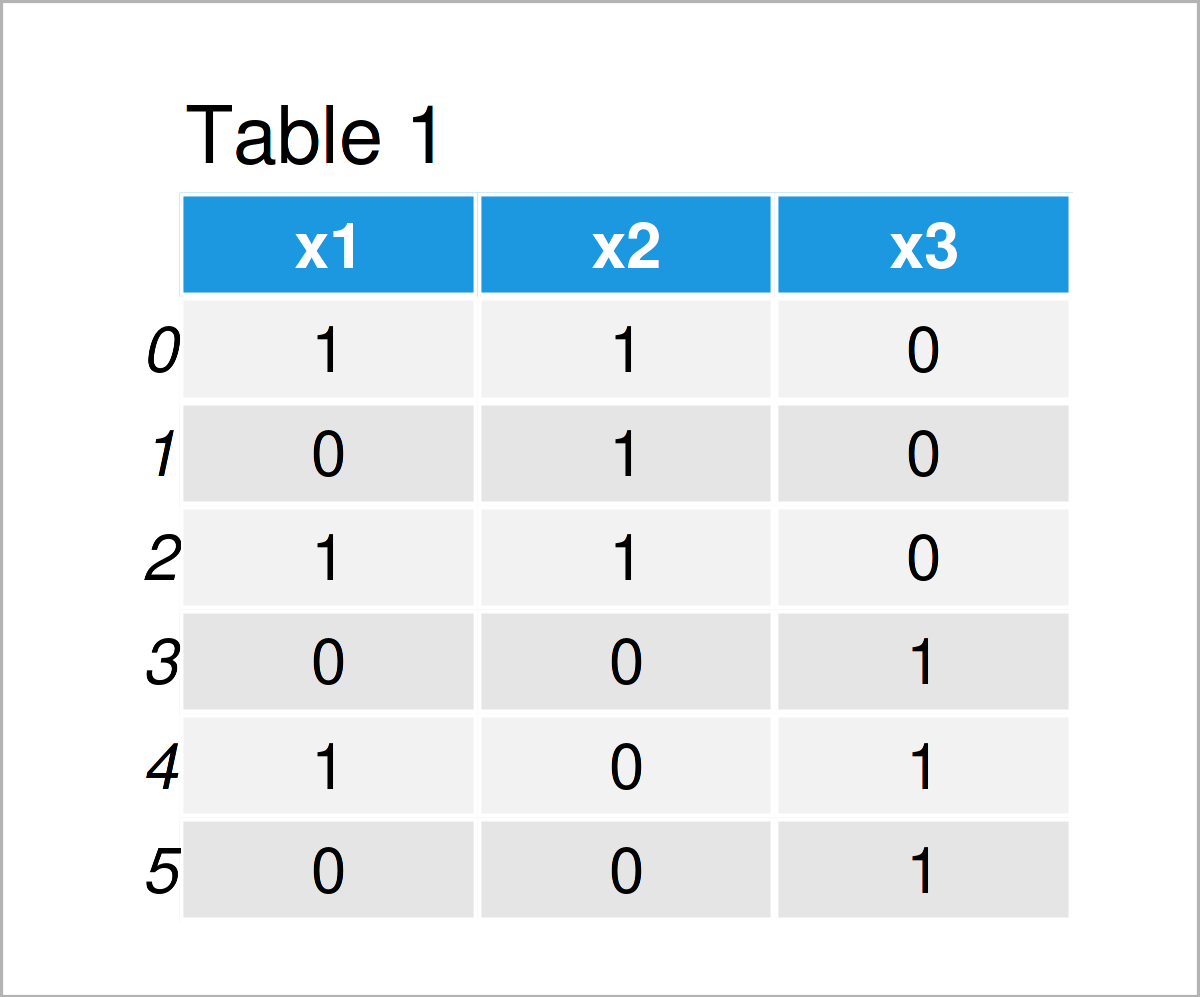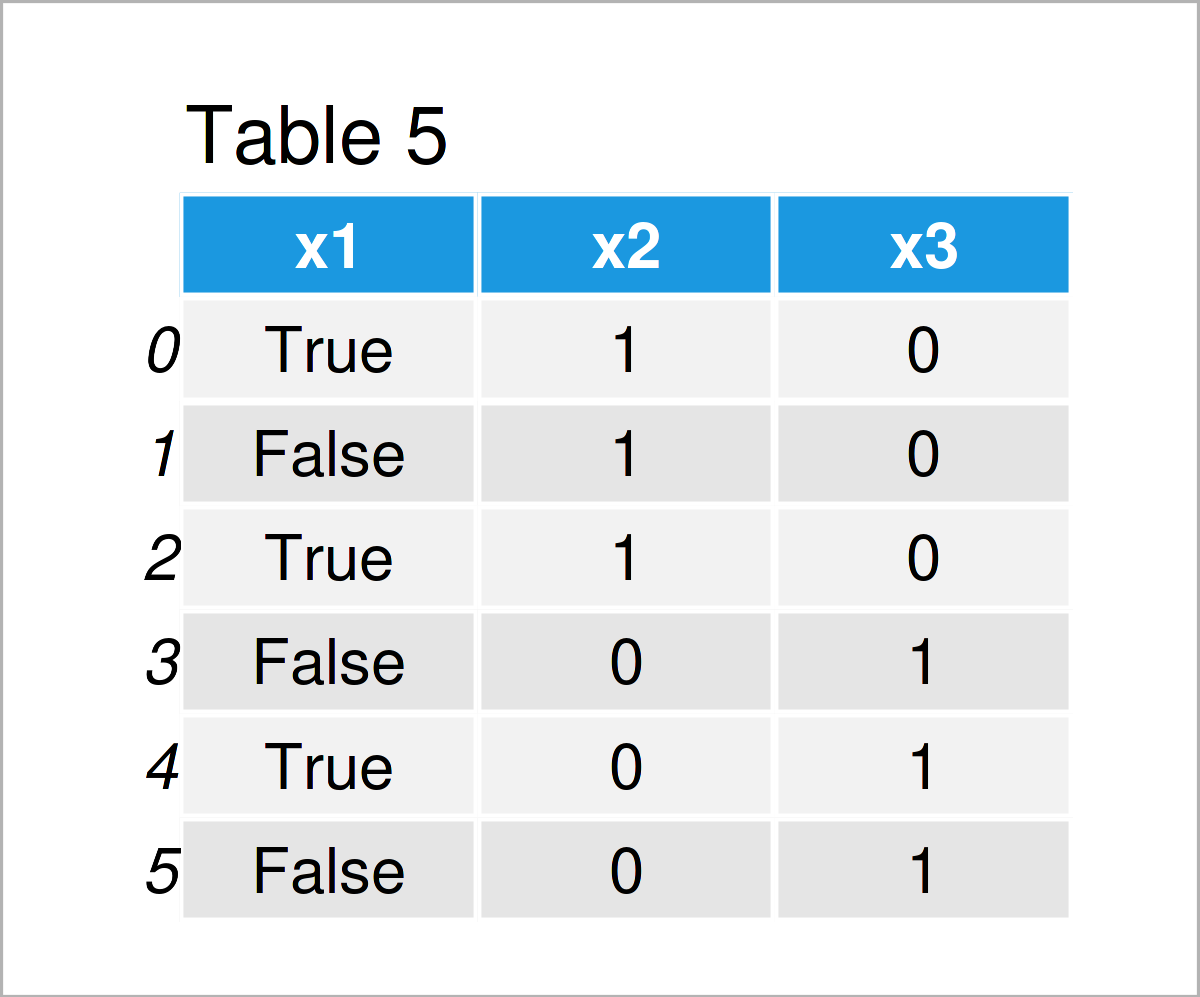# Convert 1/0 Integer Dummy to True/False Boolean in Columns of pandas DataFrame in Python (4 Examples)

In this article, I’ll demonstrate how to convert an integer column to the boolean data type in a pandas DataFrame in the Python programming language.

The article contains these content blocks:

Let’s get started.

## Exemplifying Data & Software Libraries

We first have to load the pandas library:

`import pandas as pd                                     # Load pandas library`

Furthermore, consider the example data below:

```data = pd.DataFrame({'x1':[1, 0, 1, 0, 1, 0],           # Create pandas DataFrame
'x2':[1, 1, 1, 0, 0, 0],
'x3':[0, 0, 0, 1, 1, 1]})
print(data)                                             # Print pandas DataFrame```Table 1 shows that our example pandas DataFrame consists of six rows and three columns. Note that all our columns contain integer dummy variables (i.e. the values 1 or 0).

Let’s have a look at the data types of the columns in our pandas DataFrame:

```print(data.dtypes)                                      # Check data types of columns
# x1    int64
# x2    int64
# x3    int64
# dtype: object```

At this point, all columns in our data set have the integer data type. Please note that we could apply the same syntax to variables with the float class.

Anyway, let’s jump into the examples!

## Example 1: Convert Single pandas DataFrame Column from Integer to Boolean

This section shows how to change the data type of one single column from a 1/0 integer dummy to a True/False boolean indicator.

For this task, we can apply the astype function as you can see in the following Python code:

```data_new1 = data.copy()                                 # Create copy of DataFrame
data_new1['x1'] = data_new1['x1'].astype(bool)          # Transform integer to boolean
print(data_new1)                                        # Print updated pandas DataFrame```By executing the previous Python code, we have created Table 2, i.e. a new pandas DataFrame where the first column has been transformed to the boolean class.

We can confirm this by having another look at the data types of our columns:

```print(data_new1.dtypes)                                 # Check data types of columns
# x1     bool
# x2    int64
# x3    int64
# dtype: object```

As you can see, the dtype of the variable x1 has been switched from integer to boolean.

## Example 2: Convert Multiple pandas DataFrame Columns from Integer to Boolean

In Example 2, I’ll illustrate how to transform multiple dummy columns from integer to boolean.

To accomplish this, we can use the astype function once again:

```data_new2 = data.copy()                                 # Create copy of DataFrame
data_new2 = data_new2.astype({'x2': bool, 'x3': bool})  # Transform multiple integers to boolean
print(data_new2)                                        # Print updated pandas DataFrame```The output of the previous syntax is shown in Table 3: Another pandas DataFrame where the data types of the columns x2 and x3 have been modified.

Let’s check the column classes of our data set once again:

```print(data_new2.dtypes)                                 # Check data types of columns
# x1    int64
# x2     bool
# x3     bool
# dtype: object```

The column x1 still has the integer class, but the variables x2 and x3 have been changed to the boolean data type.

## Example 3: Convert All pandas DataFrame Columns from Integer to Boolean

It is also possible to use the astype function to convert all pandas DataFrame columns from integer to boolean using the Python programming language.

In this example, I’ll demonstrate how to do that:

```data_new3 = data.copy()                                 # Create copy of DataFrame
data_new3 = data_new3.astype(bool)                      # Transform all columns to boolean
print(data_new3)                                        # Print updated pandas DataFrame```After running the previous syntax the pandas DataFrame shown in Table 4 has been created. As you can see, the values in all columns have been adjusted.

Let’s test the column classes:

```print(data_new3.dtypes)                                 # Check data types of columns
# x1    bool
# x2    bool
# x3    bool
# dtype: object```

The previous output confirms our result: All columns have been transformed to the boolean class.

## Example 4: Convert pandas DataFrame Column from Integer to Boolean Using apply() Function

In the previous examples, we have used the astype function to modify the data types of our pandas DataFrame columns.

The Python syntax below demonstrates how to use the apply function instead:

```data_new4 = data.copy()                                 # Create copy of DataFrame
data_new4['x1'] = data_new4['x1'].apply(bool)           # Transform integer to boolean
print(data_new4)                                        # Print updated pandas DataFrame```After executing the previous Python code the pandas DataFrame shown in Table 5 has been created. Similar to Example 1, we have changed only the first column.

Let’ check the column data types one last time:

```print(data_new4.dtypes)                                 # Check data types of columns
# x1     bool
# x2    int64
# x3    int64
# dtype: object```

The first column x1 has been switched from 1/0 integer to True/False boolean.

## Video & Further Resources

Would you like to learn more about the conversion of a 1/0 dummy integer column to the boolean True/False data type in a pandas DataFrame? Then you may watch the following video on my YouTube channel. I demonstrate the Python programming code of this article in the video:

In addition, you may want to read the other tutorials which I have published on this website. I have released numerous articles already:

In summary: At this point you should know how to convert a 1/0 dummy integer column to the boolean True/False data type in a pandas DataFrame in Python programming. Let me know in the comments, in case you have additional questions.

Subscribe to the Statistics Globe Newsletter